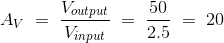# Amplifier Gain | Decibel or dB Gain

An amplifier is such an electronic device which controls a bigger electrical signal by means of a far smaller electrical signal. The output signal of this device may be voltage controlled or current controlled. An amplifier just creates a copy of input signal (control signal) but in larger magnitude with the help of external DC source. Hence, there must be an external power source in all amplifier devices. Each amplifier device has its own ability to amplify an input signal to its highest possible output magnitude. The gain of an amplifier is defined as the measure this ability of amplifying a signal. Gain is a ratio so it does not have any unit.

The ratio of output current to input current of an amplifier is defined as current gain, the ratio of output voltage to input voltage is defined as voltage gain and similarly the ratio of output power to input power of an amplifier is known as power gain of amplifier. For example if the input voltage of an amplifier is 2.5 VRMS and output voltage is 50 VRMS, the voltage gain would be,An amplifier does always not amplify in same extend as its input. It may response differently for AC and DC. Hence, another way of representing gain is the ratio of change of output signal in respect of change in input signal. That means how much change occurred in output signal for certain change in input or control signal. The gain of amplifier can be calculated easily by taking ratio of rms value of output and input signals but it is not justified technique for AC signal.

## Decibel Gain or dB Gain

Although amplifier gain does not have any unit as it is ratio of same unit signals, but if any one tries to represent the gain in logarithmic scale then it will have a unit. Generally the gain expressed in logarithmic scale will have a unit called decibel which is one tenth fraction of bel. The name of this unit that is “bel” comes from the name Alexander Graham Bell, the famous Scottish inventor who is well known for his invention of telephone systems.
Now, decibel gain is defined in terms of the common (base 10) logarithm of a power ratio that means if the conventional gain of an amplifier is Poutput / Pinput, then this gain is expressed in decibel scale as 10log(Poutput / Pinput).

If we show the table below, it will be more clearly understood.

 Gain as ratio Gain as decibel 1000 30 dB 100 20 dB 10 10 dB 1 0 dB 0.1 – 10 dB 0.01 – 20 dB 0.001 – 30 dB 0.0001 – 40 dB

### Why should we use Bel Scale or Decibel Scale to represent Gain?

The human hearing skill is logarithmic in nature. For doubling perceived intensity of sound, the sound power must be increased by 10 times. That means the gain of amplifier which controls sound intensity must have gain of 10 for doubling perceived intensity of sound which is in bel scale 1 bel and in decibel scale 10 decibel. In other words a power gain of 1 bel translates to a doubling in the perceived intensity of the sound. The perfect use of bel scale is in Richter scale which measures intensity of earthquake. 6 Richter earthquakes is 10 times more powerful than a 5 Richter earthquake, and a 4 Richter earthquake is 1/10 as powerful as 5 Richter earthquake. Logarithmic scale is also popularly used to measure concentration of hydrogen ions in a chemical solution. One pH difference in this scale means 10 folds difference in hydrogen ions concentration in a solution. It is now clear to us that where variation of gain is huge it is preferable to use logarithmic scale instead of conventional ratio scale so that tremendous range of expression can be afforded by a relatively small span of numerical values. Not only that, in cascaded amplifier the overall decimal gain is calculated by summing up individual decimal gain of amplifier instead of multiplying them as in the case of ratio gain. This makes equations and related calculations simpler and easier.
Suppose one amplifier has gain of 3 and another has 5 and these two amplifiers are connected in cascaded manner. Overall gain of the system is 3 × 5 = 15. Now in logarithmic or dB scale these gain are 10 log3 = 4.77 dB and 10 log 5 = 6.99 dB respectively. In decibel scale this overall gain is 10 log15 = 11.76 dB which is nothing but 4.77 + 6.99. Thus we have seen how overall gain can be calculated by adding individual gain instead of multiplying them.

Want To Learn Faster? 🎓
Get electrical articles delivered to your inbox every week.
No credit card required—it’s 100% free.